×#### Thank you for registering.

One of our academic counsellors will contact you within 1 working day.

Click to Chat

1800-1023-196

+91-120-4616500

CART 0

• 0

MY CART (5)

Use Coupon: CART20 and get 20% off on all online Study Material

ITEM
DETAILS
MRP
DISCOUNT
FINAL PRICE
Total Price: Rs.

There are no items in this cart.
Continue Shopping• Complete JEE Main/Advanced Course and Test Series
• OFFERED PRICE: Rs. 15,900
• View Details

```Chapter 3: Rationalisation Exercise – 3.1

Question: 1

Simplify each of the following:Solution: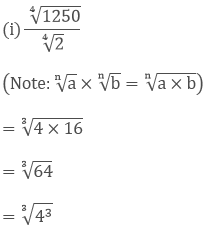= (43)1/3

= 4(3 × 1/3)

= 41

= 4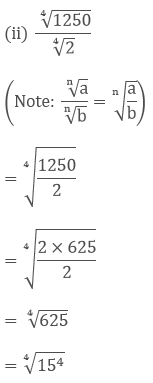= 15(4×1/4)

= 15

Question: 2

Simplify the following expressions: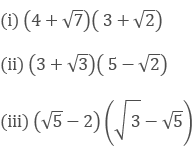Solution:Question: 3

Simplify the following expressions:Solution: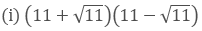As we know, (a + b) (a - b) = (a2 − b2)

So, 112 - 11

121 - 11 = 110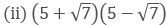As we know, (a + b) (a - b) = (a2 − b2)

So, 52 - 7

25 - 7 = 18As we know, (a + b) (a - b) = (a2 − b2)= 6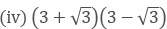As we know, (a + b) (a - b) = (a2 − b2)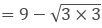= 6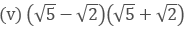As we know, (a + b) (a - b) = (a2 − b2)= 5 - 2

= 3

Question: 4

Simplify the following expressions:Solution: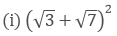As we know, (a + b)2 = (a2 + 2 × a × b + b2)As we know, (a - b)2  = (a2 − 2 × a × b + b2)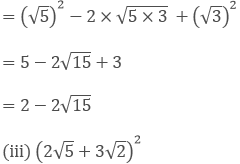As we know, (a + b)2 = (a2 + 2 × a × b + b2)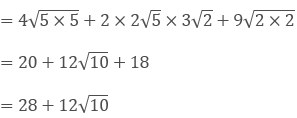```### Course Features

• 728 Video Lectures
• Revision Notes
• Previous Year Papers
• Mind Map
• Study Planner
• NCERT Solutions
• Discussion Forum
• Test paper with Video Solution